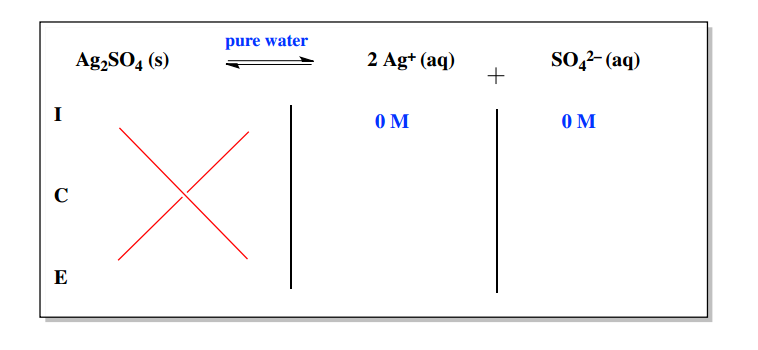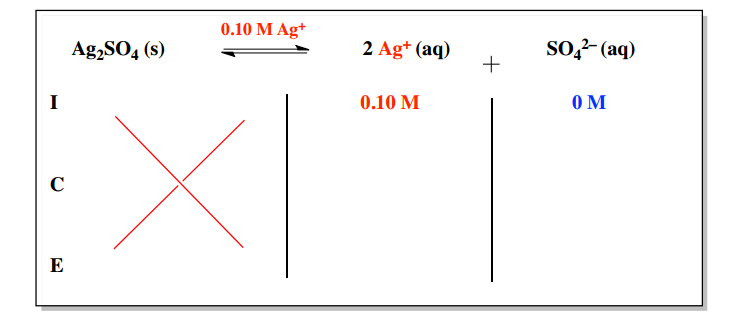Clutch Prep is now a part of Pearson
Ch.16 - Aqueous Equilibrium WorksheetSee all chapters

# Ksp

See all sections
Sections
Buffer
Acid and Base Titration Curves
Weak Acid Strong Base Titrations
Weak Base Strong Acid Titrations
Strong Acid Strong Base Titrations
Titrations of Diprotic and Polyprotic Acids
Ksp
Henderson Hasselbalch Equation (IGNORE)

Associated with any ionic compound is the solubility product constant, which measures how soluble the compound will be in a solvent.

###### Molar Solubility

Concept #1: Understanding the Solubility Product Constant.

Transcript

Hey guys, in this new video, we’re going to take a look at a new concept – the solubility product constant. We’re used to so many Ks up to now. We've seen Ka, we’ve seen Kb. But now we're going to be dealing with Ksp which we’re going to refer to as our solubility product constant. We're going to say here that solubility was the maximum amount of solute that could successfully be dissolved in a solvent. Remember, another name for solubility is molarity. Another name for molarity is concentration. Solubility, molarity, and concentration – all three, different words but all mean the same exact thing. How much solute can I get to dissolve in my given solvent.
Now we're going to say in this section we’re going to learn about associated with any type of solid. Any solid is the Ksp value. We’re going to say the Ksp’s value stands for what we've been saying – the solubility product constant. We’re going to say the larger our Ksp value is, then the more soluble the solid is in its given solvent. We're going to say the smaller our Ksp value, then the less soluble the solid is in its given solvent.
Ksp is just the measurement of solubility, how soluble is your solid. If you’re ever giving Ksp for a compound, you should know automatically since this compound is given a Ksp value, the compound has to be a solid.

The general rule is that the greater the solubility product constant for a compound then the more soluble the compound.

Example #1: Consider the following compounds. Which has the highest concentration of OH- ions?
a) Co(OH)2         Ksp = 1.3 x 10-15

b) Cu(OH)2         Ksp = 2.2 x 10-20

c) Ni(OH)2          Ksp = 6.0 x 10-16

d) Fe(OH)2         Ksp = 4.1 x 10-15

e) Zn(OH)2         Ksp = 3.0 x 10-16

Transcript

Now we're going to say example 1. Consider the following compounds. Which has the highest concentration of OH- ions? What we should realize here is that all of them have two OH- involved. Because they all have the same number of OH-, that means that is not a deciding factor, so just ignore that. That means just look at the Ksp value. We're going to say the one that has the highest Ksp value would be the most soluble, which means it breaks up into the most ions. The one that breaks up into the most ions would have the highest concentration of OH- ions. All we got to do here is look to see which Ksp is the biggest. Here we have two that are to the negative 15. But this one is 4.1 and this one is just 1.3. So, D would be our correct answer.
Another way your professor could have worded this is remember, associated with OH- ions is basicity. The more OH- you have, the more basic you are. I could have worded this as which is the most basic. You should have read into that and said, “Okay, he means most basic so that means who has the highest amount of OH-.” I could have also said least acidic, which of the following is least acidic, which would have mean who has the lowest amount of H3O+ ions or who has the highest concentration of OH- ions, different ways. Three different ways that ask the same exact question. Remember, because all of them had two OH-, we didn't have to look at the number of ions. We only looked at the KSP. If they had different number of OH- ions, then we’d have to do calculations in order to find their molar solubility which is x. We'll learn how to do that a little bit later on.

Example #2:  The solubility of silver sulfate, Ag2SO4, is 0.025 M at 25oC. Calculate its solubility product constant, Ksp

Whenever the question refers to the solubility then that value represents our “x” variable.

Example #3:  Find the solubility of CoCl3 (Ksp = 2.8 x 10-13) in:

a) pure water

Whenever we deal with the solubility product constant we use an ICE Chart and since the reactant will always be a solid then we can ignore it.If our compound dissolves in a solution with similar ions then the Common Ion Effect takes place.Example #4:  Find the solubility of CoCl3 (Ksp = 2.8 x 10-13) in:

b) 0.20 M NaCl.

Example #5: What is the molar solubility of Fe(OH)3 (s) in a solution that is buffered at pH 3.50 at 25 oC? The Ksp of Fe(OH)3 is 6.3 x 10–38 at 25 oC.

Example #6: Find the pH of a saturated solution of Aluminum hydroxide, Al(OH)3. The Ksp of Al(OH)3 is 1.9 x 10-10

Example #7: Will a precipitate form when 0.150 L of 0.100 M Pb(C2H3O2)2 and 0.100 L of 0.20 M KCl are mixed? The Ksp value of PbCl2 is 1.2 x 10-5

Example #8: Two flasks containing 7.12 x 10-6 M silver nitrate, AgNO3, and 8.33 x 10-4 M strontium bromide, SrBr2 respectively, are mixed together into a larger flask. If the Ksp of silver bromide, AgBr, is 5.35 x 10-13 what will happen?

1. A precipitate of Sr(NO3)2 will form.
2. The solution is saturated but will remain clear.
3. The solution is not saturated and no precipitate will form.
4. A precipitate of silver bromide will form, with silver and bromide ions as well.
5. A precipitate of silver bromide will form.

Example #9: A saturated solution of AlCl3 contains 5.15 x 10-4 M chloride ions. What is the solubility product constant, Ksp?

Example #10: Molar Solubility of Ions

Example #11: The solubility of CaF2 is 53.2 g/mL at 25 oC. Calculate its solubility product constant, Ksp.

Example #12: The concentration of chloride and iodide ions present in the solution are [Cl ] = 0.073 M and [I ] = 0.060 M. The use of silver, Ag, can be used to precipitate these two ions. If the Ksp value of AgCl is 1.77 x 10-10 and AgI is 8.51 x 10-17.

a) Which ion will precipitate out first?

b)  At what concentration will this ion from Part A begin to precipitate out?